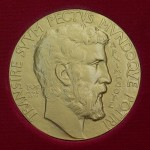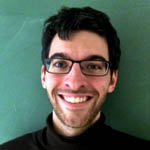# A Fields Medal at UCL: Klaus Roth

Klaus Roth and his workBe proud if you are studying Mathematics at UCL! Looking back, we have numerous famous alumni who later gained significant achievements in their field.  One of them is Klaus Roth, who was once a research student at UCL, and later was a lecturer and professor at the university, during which time he won the Fields Medal.

If you haven’t heard of the Fields Medal, it is seen as the equivalent of the ‘Nobel Prize’ in Mathematics (although unfortunately it has a much lower monetary reward) and is awarded every four years by the International Mathematical Union.  The award is given to a maximum of four mathematicians each time, all of whom must be under the age of 40 and have made a great contribution to the development of Mathematics.  Roth won the Medal in 1958, when he was 33 years old and still a lecturer at UCL (show more respect to your lecturers … you never know!), for having “solved in 1955 the famous Thue-Siegel problem concerning the approximation to algebraic numbers by rational numbers and proved in 1952 that a sequence with no three numbers in arithmetic progression has zero density (a conjecture of Erdös and Turàn of 1935).”The Fields Medal

Born in Breslau, Prussia (now Wroclaw in Poland) in 1925, Roth moved to England at a young age.  He attended St. Paul’s School in London, and then went to Cambridge for his BA degree.  After graduating in 1945, he worked for a brief time at Gordonstoun School in Scotland, before coming back to London and starting his research in 1946 under the supervision of Theodor Estermann.  Two years later he was awarded his master’s degree and two years after that, in 1950, he achieved his PhD.  He was working at the same time as an assistant lecturer and later he became a lecturer, a reader, and eventually a professor at UCL in 1961.

In 1966, Roth was offered the post of chair of Pure Mathematics at Imperial College, which he accepted and retained until his retirement in 1988.  He has won numerous awards on top of the Fields Medal, including the De Morgan Medal from the London Mathematical Society (LMS) in 1983 and the Sylvester Medal from the Royal Society in 1991.  Roth is currently 89 years old, and residing in the north of Scotland.

### Roth’s WorkThe Fields Medal

Klaus Roth specialised in a branch of mathematics known as number theory, and most particularly in diophantine approximations, which look at approximating real numbers by rational numbers (those that can be expressed in the form $p/q$, where $p$ and $q$ are integers). The work for which he won the Fields Medal in 1958 dealt with approximating a particular class of real numbers known as algebraic numbers: those that are the solutions of finite polynomials with rational coefficients. For example, the irrational number $\sqrt{2}$ is an algebraic number as it is a solution to the equation $x^2 – 2 = 0$; whilst such famous numbers as $\pi$ and $e$ are not the solution of any such polynomial and hence are called transcendental.

When it came to approximating algebraic numbers, people knew that there were infinitely many rational numbers $p/q$ such that

$$\left| \frac{p}{q} – a \right|< \frac{1}{q^2}, \quad (1)$$

where $a$ is an algebraic number. You can show this by writing $a$ as a continued fraction,

$$a =\frac{1}{n_1 + \frac{1}{n_2 + \frac{1}{n_3 + \dots}}}.$$

However, the question was whether we could do better: for a given algebraic number, could we find an exponent $x$ greater than 2 such that there are infinitely many rational numbers (again of the form $p/q$) that approximate the algebraic number with an error less than $q^{-x}$? Or, expressing this more mathematically, for a given algebraic number $a$, let $\mu(a)$ be the upper bound of the exponents $x$ such that there exist infinitely many rational numbers $p/q$ satisfying

$$\left|\frac{p}{q} – a \right| < \frac{1}{q^x}.$$

The question is then to find $\mu$.

Because of the result in (1), $\mu(r) \geq 2$. In 1844, Liouville bounded $\mu$ from above, proving that if $a$ was the solution to a polynomial of degree $d$ (the highest power of the unknown in the equation is $d$), then $\mu(a) \leq d$. So there were certainly only finitely many rational numbers that approximated $a$ with an error better than $q^{-d}$. A range of $\mu$ between 2 and $d$ was, however, not good enough and various mathematicians narrowed it down before Roth’s decisive contribution. In 1908, Thue showed that $\mu(a) \leq d/2 + 1$; in 1921, Siegel brought the upper limit down to $2\sqrt{d}$; in 1947, Dyson improved it still further to around $\sqrt{2d}$. And then, in 1955, Roth showed that $\mu(r)$ was actually equal to 2.

In other words, if we want to improve the accuracy of our estimation to be better than $1/q^2$, then there are only finitely many rational numbers able to achieve this. In the words of Davenport, who presented Roth with the Fields medal, the theorem “settles a question which is both of a fundamental nature and of extreme difficulty. It will stand as a landmark in mathematics for as long as mathematics is cultivated.”

• With contributions from Pietro Servini.Jessie is a first-year undergraduate studying mathematics at UCL.Pietro is interested in history and sport. He also happens to be doing a PhD in fluid dynamics at UCL. If he can combine any two of the three it makes him a happy man.